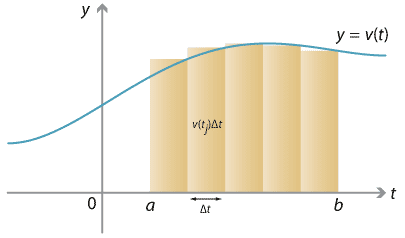## Content

### Velocity–time graphs

Let us now return to our original motivating question with the bike and speedometer. Let $$v(t)$$ denote your velocity on your bike at time $$t$$, and we now calculate your total change in position between time $$t=a$$ and $$t=b$$. As mentioned earlier, if $$v(t)$$ is a constant function, and so you are travelling at constant velocity, then your total change in position, or displacement, is $$\Delta s = v \; \Delta t$$.

Our idea of estimating your distance travelled by breaking your trip up into 1-minute intervals is nothing more than an area estimate. We may divide the time interval $$[a,b]$$ into $$n$$ subintervals $$[t_0, t_1], [t_1, t_2], \dots, [t_{n-1}, t_n]$$, each of width $$\Delta t = \frac{1}{n} (b-a)$$. We can estimate your velocity over the interval $$[t_{j-1}, t_j]$$ by the constant $$v(t_j)$$ (i.e., using the right-endpoint estimate); over this interval of time, you travelled a distance of approximately $$v(t_j) \; \Delta t$$. The total distance travelled, then, is approximately

$\sum_{j=1}^n v(t_j) \; \Delta t.$Detailed description of diagram

$\text{The right-endpoint estimate for area under} \ y = v(t) \ \text{estimates displacement.}$

In the limit, as we take more and more shorter and shorter time intervals, we obtain the exact change in position (displacement) as,

$\int_a^b v(t) \; dt.$

Therefore, the total displacement is the area under the velocity–time graph $$y = v(t)$$.

Note that this integrand is expressed as a function of $$t$$, and $$dt$$ indicates that we integrate over~$$t$$. If we used $$v(x)$$ and $$dx$$ instead, the meaning would be identical:

$\int_a^b v(t) \; dt = \int_a^b v(x) \; dx.$

The variable $$t$$ (or $$x$$) just indexes the values of the integrand; it is only used for the integral's own bookkeeping purposes. It is known as a dummy variable, analogous to the dummy variable in summation notation, like the $$j$$ in $$\sum_{j=1}^5 j^2$$.

Next page - Content - Areas above and below the axis